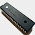## Saturday, June 30, 2012

### Cubic Formula - BASIC Program (HP 71B)

Finding the roots of the cubic equation ax^3 + bx^2 + cx + d = 0 can be a challenge. Even with a cubic formula, multiple and sometimes complicated steps can be involved.

Source:

In this program my general strategy is:
1. Find the first root X1.
2. Use X1 to invoke synthetic division to reduce the cubic equation to a quadratic equation.
3. Use a modified quadratic equation to find the other two roots. The link provides good formula for finding the remaining two roots.

I assume that the coefficients a, b, c, and d are real numbers. This guarantees that one of the roots (X1) is a real number.

Program: Cubic Formula in BASIC
6/30/2012 - 533 bytes

Equation:

A*X^3 + B*X^2 + C*X + D = 0
Solve for X

Formula breakdown (General):

Let P = 2*B^3 - 9*A*B*C + 27*A^2*D
and Q = B^2 - 3*A*C

Let X1, X2, and X3 be the roots and:

X1 = -B/(3*A) - 1/(3*A)*( .5*(P+√(P^2-4*Q^3)))^(1/3) + (.5*(P-√(P^2-4*Q^3)))^(1/3) )

Once X1 is found, symmetric division is used to reduce the cubic equation to a quadratic equation. The roots X2 and X3 are now found.

Let J = -B - A*X1
and K = B^2 - 4*A*C - 2*A*B*X1 - 3*A^2*X1^2

X2 and X3 come from the formula:

(J ± √(K)) / (2*A)

If A, B, C, and D are real numbers (no of the coefficients has an imaginary part), X1 will be a real root. X2 and X3 will either be two real roots or conjugate complex numbers.

Notes that the HP 71B has no native complex mode, so this program uses several work arounds. In addition:

1. I could not take odd number roots of negative numbers. Work around for Cube Root: ABS(X)^(1/3)*SGN(X)
2. I use a trigonometric work around when .25 * (P^2 - 4*Q^3) 3. Notation wise, SQR stands for square root, ABS stands for absolute value, and ANGLE is the angle function ( atan2(x,y) ).
4. All the used variables are "destroyed" to clear them and assign them to real numbers.

Variables:
A, B, C, D: Coefficients
A1, P, Q, P1, P2: Used in interim calculations
X1: Root X1
The other two roots are:
X2, X3: Real roots X2, X3
or
S, T: Complex Conjugates of X2 and X3 (S ± Ti)

Program - HP 71B Basic Computer:

10 DISP 'CUBIC'
15 DESTROY A,B,C,D,P,Q,A1,P1,X1,X2,X3,P2,S,T
20 INPUT "A,B,C,D"; A,B,C,D
30 P = 2*B^3 - 9*A*B*C + 27*A^2*D
32 Q = B^2 - 3*A*C
34 A1 = .25*(P^2 -4*Q^3)
36 IF A1<0 THEN 48

38 P1 = .5*P+SQR(A1)
40 P2 = .5*P-SQR(A1)
42 X1 = -B/(3*A) - 1/(3*A)*(ABS(P1)^(1/3)*SGN(1/3) + ABS(P2)^(1/3)*SGN(P2))
44 GOTO 60

48 P1 = SQR((.5*P)^2 + ABS(A1))
50 P2 = ANGLE(.5*P, SQR(ABS(A1)))
52 X1 = -B/(3*A) - 2/(3*A) * (P1^(1/3)*COS(P2/3))

60 DISP 'X1=';X1
62 PAUSE
64 J=-B-A*X1
66 K=B^2-4*A*C-2*A*B*X1-3*A^2*X1^2
68 IF K>=0 THEN 70 ELSE 90

70 X2=(J+SQR(K))/(2*A)
72 X3=(J-SQR(K))/(2*A)
74 DISP 'X2='; X2
76 PAUSE
78 DISP 'X3='; X3
80 END

90 S=J/(2*A)
92 T=SQR(ABS(K))/(2*A)
94 DISP 'REAL=';S
96 PAUSE
98 DISP 'IMAG=';T

-----------------------------------------------------
Test runs:
3x^3 + 3x^2 - 3x + 6 = 0

X1 = -1.9999999999 (really -2)
REAL = .49999999999
IMAG = .86602403772
(.5 ± i √.75)

x^3 + 4x^2 + x - 3 = 0

X1 = -3.46050487001
X2 = .69928148275
X3 = -1.23912327826

-2x^3 - x + 5 = 0
X1 = 1.23477282504
REAL = -.61738641252
IMAG = -1.2819898392

x^3 - 2x^2 - 5x + 6 = 0
X1 = -1.9999999999 (really -2)
X2 = 3
X3 = .99999999985 (really 1)

A Triple Root: x^3 - 3x^2 + 3x - 1 = 0
X1 = 1
X2 = 1
X3 = 1

This blog is property of Edward Shore. © 2012

Updated 7/1/2012 - thanks to the awesome people the MoHPC. (www.hpmuseum.org)

#### 1 comment:

1.Can you please post what is the value of variables (P, Q, A1, P1, P2) in test run #2 (x^3 + 4x^2 + x - 3 = 0) because i don't get x1 = -3.460... but x1 = -3.735... and is P2(ANGLE = (x,y) in DEG or RAD ?
I want to run this program on my casio fc 4800P .
Thank you

### Retro Review: Radio Shack EC-2024

Retro Review:  Radio Shack EC-2024 Quick Facts Company:  Radio Shack Years in Production: Around 1994 Original Price: \$24.99 Bat...